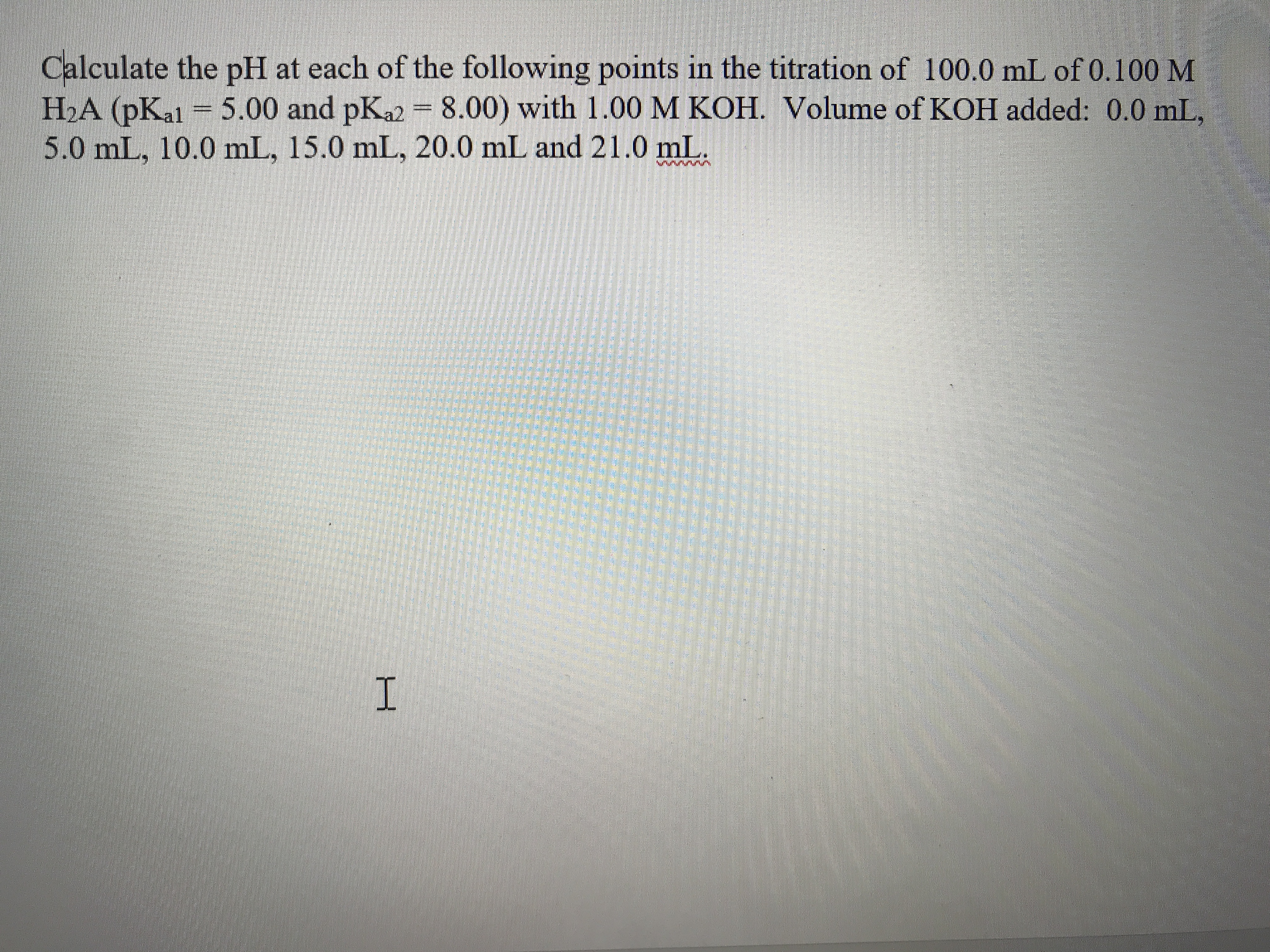# Calculate the pH at each of the following points in the titration of 100.0 mL of 0.100 M H2A (pKal 5.00 and pKa2 8.00) with 1.00 M KOH. Volume of KOH added: 0.0 mL 5.0 mL, 10.0 mL, 15.0 mL, 20.0 mL and 21.0 mL I

Question

Calculate the pH at each of the following points in the titration of  100.0 mL of 0.100 M H2A (pKa1 = 5.00 and pKa2 = 8.00) with 1.00 M KOH.  Volume of KOH added:  0.0 mL,  5.0 mL, 10.0 mL, 15.0 mL, 20.0 mL and 21.0 mL.help_outlineImage TranscriptioncloseCalculate the pH at each of the following points in the titration of 100.0 mL of 0.100 M H2A (pKal 5.00 and pKa2 8.00) with 1.00 M KOH. Volume of KOH added: 0.0 mL 5.0 mL, 10.0 mL, 15.0 mL, 20.0 mL and 21.0 mL I fullscreen

### Want to see this answer and more?

Experts are waiting 24/7 to provide step-by-step solutions in as fast as 30 minutes!*

*Response times vary by subject and question complexity. Median response time is 34 minutes and may be longer for new subjects.
Tagged in
ScienceChemistry

### General Chemistry